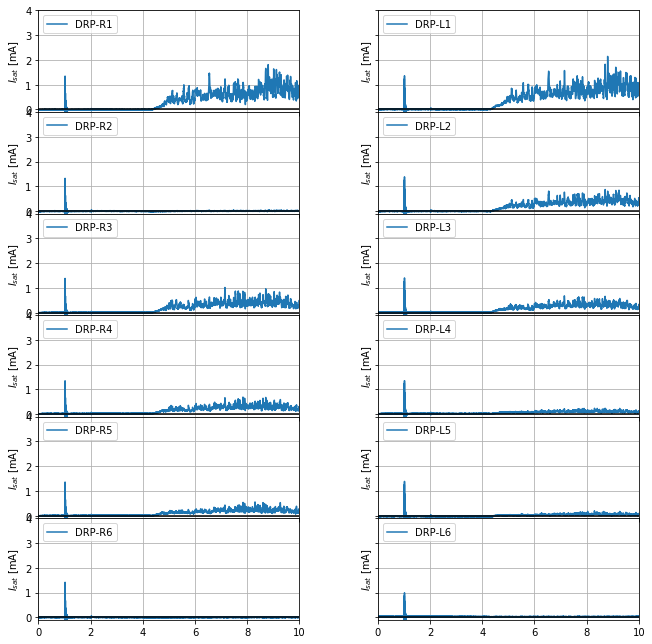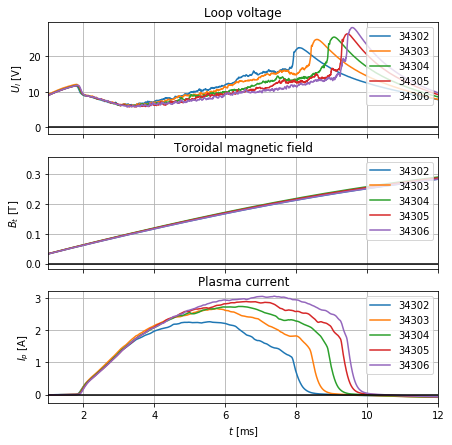# Double rake probe spotlight¶

The following scripts introduces basic aspects of the double rake probe measurements.

## The double rake probe diagnostic¶

This is the double rake probe:Its wiki page is located here. The double rake probe has two rows of pins ("rakes"), out of which the first 6 in each row are connected to the data acquisition system. The pin signals are called DRP-R1 to DRP-R6 and DRP-L1 to DRP-L5.

Like any Langmuir probe, the rake probe pins can be electrically insulated (measuring the floating potential $V_f$), biased to a negative voltage -100 V (measuring the ion saturated current $I_{sat}$), their biasing voltage may be swept (measuring $V_f$, $I_{sat}$ and the electron temperature $T_e$ if the $I$-$V$ characteristic is fitted with an exponential function) or they can perform other measurements. During this campaign, all the pins measure the ion saturated current $I_{sat}$. This can be processed to gain information about the plasma turbulent transport.

## Import basic libraries¶

To visualise and process the double rake probe data, first we import basic libraries, Numpy and Matplotlib.

In :
%matplotlib inline
import numpy as np
import matplotlib.pyplot as pl


## Load double rake probe data¶

The data directory of the double rake probe is http://golem.fjfi.cvut.cz/shots/{shot}/DASs/DoubleRake/. We write a function to download the data.

In :
from urllib.error import HTTPError # recognise the error stemming from missing data
import pandas as pd # for reading csv files

#Define an exception which will be raised if the data is missing and stop the notebook execution
class StopExecution(Exception):
def _render_traceback_(self):
pass

def get_data(shot, identifier):
URL = "http://golem.fjfi.cvut.cz/shots/{shot}/Diagnostics/DoubleRakeProbe/{identifier}.csv"
url = URL.format(shot=shot, identifier=identifier)
try:
df = pd.read_csv(url, names=['time', identifier], index_col='time')
except HTTPError:
raise StopExecution
t = np.array(df.index)
data = np.transpose(np.array(df))
return t, data/46


Notice that in the last line, the data values are divided by 46. This is the constant which converts the raw DAS signal to the ion saturated current in A.

## Plot all the signals from the double rake probe pins¶

We load the double rake probe data for the current discharge and plot them.

In :
#Load the signals of all the rake probe pins
shot = 0
DRP_R = []
DRP_L = []
for i in range(1, 7):
t, data = get_data(shot, 'DRP-R%i' % i)
DRP_R.append(data)
for i in range(1, 7):
t, data = get_data(shot, 'DRP-L%i' % i)
DRP_L.append(data)
DRP_R = np.array(DRP_R)
DRP_L = np.array(DRP_L)

#Plot the rake probe pin signals
fig, axs = pl.subplots(6, 2, num=('All rake probe signals in the current discharge'), figsize=(9,9),
sharex=True, sharey=True)
for i in range(0, 6):
axs[i, 0].plot(t*1000, DRP_R[i]*1000, label='DRP-R%i' % (i+1))
for i in range(0, 6):
axs[i, 1].plot(t*1000, DRP_L[i]*1000, label='DRP-L%i' % (i+1))
fig.tight_layout()
for i in range(6):
for j in range(2):
axs[i,j].legend(loc=2)
axs[i,j].grid(True)
axs[i,j].set_xlim(0, 10)
axs[i,j].set_ylim(-0.1, 4)
axs[i,j].axhline(c='k')
axs[i,j].set_ylabel('$I_{sat}$ [mA]')
pl.savefig('icon-fig')## Define a double rake probe campaign¶

The rake probe typically measures a whole profile on a shot-to-shot basis. During this measurement, the same discharge is repeated again and again while the probe is moved to new positions. Assuming the plasma is the same in all the discharges, the probe collects the profile of the ion saturated current.

In the following, we load and print the campaign discharges and the rake probe position in these discharges. The probe position is read on a scale on the probe manipulator and it denotes the vertical distance from the midplane $Z$.

In :
# Later these arrays will be passed to the script as parameters
shots = np.array([34302, 34303, 34304, 34305, 34306])
Z = np.array([0.1, 0.09, 0.08, 0.07, 0.06]) #first pin distance from the midplane in m

print('Shot    Distance of the first pin from the midplane (Z)')
for i in range(shots.size):
print('%i   %i cm' % (shots[i], 100*Z[i]))

Shot    Distance of the first pin from the midplane (Z)
34302   10 cm
34303   9 cm
34304   8 cm
34305   7 cm
34306   6 cm


## Check discharge reproducibility in the campaign¶

To ensure that the shots within the campaign are identical, we plot their loop voltages, magnetic fields and plasma currents over each other. First we write a function which loads the basic diagnostics data.

In :
def get_basic_diag_data(shot):
'''
Return the standard diagnostics data for given shot:
time axis [s], loop voltage U_l [V], toroidal magnetic field B_t [T] and plasma current I_p [T].
'''
url = ( 'http://golem.fjfi.cvut.cz/shots/{}/Diagnostics/BasicDiagnostics/Analysis_dir/'
'basig_diagnostics_processed.csv'.format(shot) )
t = np.array(df.index) * 1e-3 #ms to s
data = np.transpose(np.array(df))
data[3:4] *= 1e3 #kA to A
return t, data, data, data


Next, we write a function which plots the basic diagnostics in our campaign over one another. If the loop voltage $U_l$, torodial magnetic field $B_t$ and plasma current $I_p$ are the same in all the discharges, the campaign may serve as a good measurement of the ion saturated current profile.

In :
def campaign_basic_diagnostics():
#Prepare figure
fig, axs = pl.subplots(3, 1, num=('Campaign basics diagnostics'), figsize=(7,7), sharex=True)

#Load and plot the basic diagnostics
for shot in shots:
t, Ul, Bt, Ip = get_basic_diag_data(shot)
axs.plot(t*1000, Ul, label=str(shot))
axs.plot(t*1000, Bt, label=str(shot))
axs.plot(t*1000, Ip, label=str(shot))

#Label the plot
labels = ['$U_l$ [V]', '$B_t$ [T]', '$I_p$ [A]']
names = ['Loop voltage', 'Toroidal magnetic field', 'Plasma current']
for i in range(3):
axs[i].legend(loc=1)
axs[i].set_xlim(1, 12)
axs[i].grid(True)
axs[i].set_ylabel(labels[i])
axs[i].set_title(names[i])
axs[i].axhline(c='k')
axs.set_xlabel('$t$ [ms]')
return

campaign_basic_diagnostics()In [ ]: